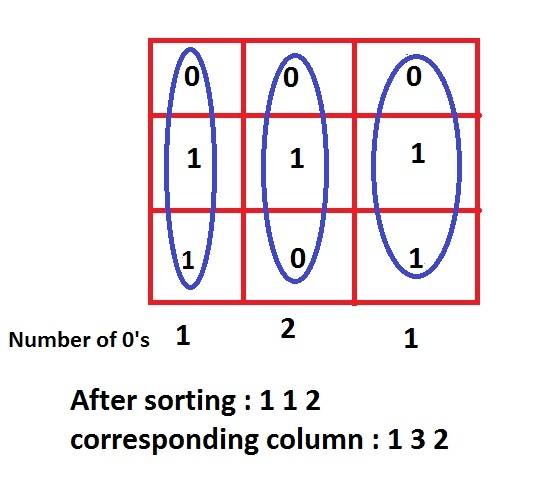# Print index of columns sorted by count of zeroes in the Given Matrix in C Program.

Given an array of size NxM where N number of rows and M number of columns, and the task is to print the number of zeroes in every column of a corresponding matrix after performing sort operation on the basis of number of zeros present in any column.

For example if the 1st column contain 1 zeros and 2nd column doesn’t contain any number of zeros and 3rd column contain 2 zeroes then the result should be − 3 1 2.

## Example

Input:
0 0 0
1 1 1
1 0 1
Output: 1 3 2

## ExplanationNote − the matrix is considered started from index 1.

## Example

#include <bits/stdc++.h>
#define row 3
#define col 3
using namespace std;
void sorting(int arr[row][col]){
vector<pair<int, int> >count_zero;
for (int i = 0; i < col; i++){
int count = 0;
for (int j = 0; j < row; j++){
if (arr[j][i] == 0)
count++;
}
count_zero.push_back(make_pair(count, i));
}
sort(count_zero.begin(), count_zero.end());
for (int i = 0; i < col; i++)
cout<< count_zero[i].second + 1 << " ";
}
int main(){
int array[row][col] = {
{ 0, 0, 0 },
{ 1, 1, 1 },
{ 1, 0, 1 }
};
cout<<"sorted order of zeroes count is : ";
sorting(array);
return 0;
}

## Output

if we run the above program then it will generate the following output

sorted order of zeroes count is : 1 3 2